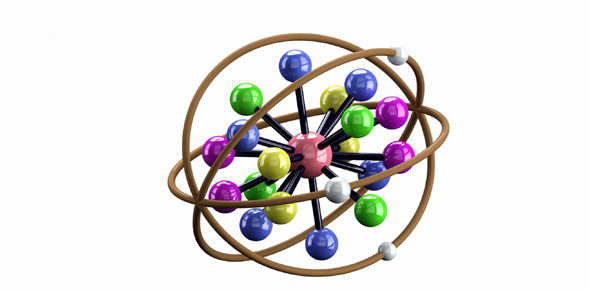# 2-1. Atoms, Molecules And Ions

Approved & Edited by ProProfs Editorial Team
At ProProfs Quizzes, our dedicated in-house team of experts takes pride in their work. With a sharp eye for detail, they meticulously review each quiz. This ensures that every quiz, taken by over 100 million users, meets our standards of accuracy, clarity, and engagement.
| Written by QuizzesAndTests
Q
QuizzesAndTests
Community Contributor
Quizzes Created: 2 | Total Attempts: 2,075
Questions: 10 | Attempts: 1,142SettingsAs we continue to learn more about atoms and the chemical changes they undergo it is important to ensure we are on our feet regarding what we have already covered. The quiz below will help you know what you understand on atomic mass, number and structure. Give it a try and keep on revising.

• 1.

### . What is the identity of element X?

• A.

Silicon

• B.

Carbon

• C.

Oxygen

• D.

B. Carbon
Explanation
The correct answer is Carbon because the question asks for the identity of element X, and Carbon is the only option provided that could potentially be element X. The other options (Silicon and Oxygen) are not relevant to the question.

Rate this question:

• 2.

### True or false: The identity of an element is determined by the number of neutrons.

• A.

True

• B.

False

B. False
Explanation
The identity of an element is determined by the number of protons in its nucleus, which is known as the atomic number. The number of neutrons can vary within an element, resulting in different isotopes of the same element. Therefore, the statement that the identity of an element is determined by the number of neutrons is false.

Rate this question:

• 3.

### True or false: The identity of an element is determined by the number of protons.

• A.

True

• B.

False

A. True
Explanation
The identity of an element is determined by the number of protons because the number of protons in an atom is unique to each element. This number is known as the atomic number and it defines the element's properties and place in the periodic table. Changing the number of protons would result in a different element altogether. Therefore, the statement "The identity of an element is determined by the number of protons" is true.

Rate this question:

• 4.

### Check all of the values that can be determined given this atomic notation:

• A.

Atomic number

• B.

Mass number

• C.

Number of neutrons

• D.

Number of protons

A. Atomic number
B. Mass number
C. Number of neutrons
D. Number of protons
Explanation
The atomic number is the number of protons in an atom, which can be determined from the atomic notation. The mass number is the sum of the number of protons and neutrons, so it can also be determined. The number of neutrons can be calculated by subtracting the atomic number from the mass number. Therefore, all of the values listed can be determined given the atomic notation.

Rate this question:

• 5.

### True or false: The atomic number is the same as the number of protons.

• A.

True

• B.

False

A. True
Explanation
The atomic number of an element represents the number of protons in the nucleus of its atom. Since protons carry a positive charge, the atomic number also indicates the number of electrons in a neutral atom. Therefore, the statement "The atomic number is the same as the number of protons" is true because the atomic number uniquely identifies an element based on the number of protons in its atom.

Rate this question:

• 6.

### An element has a mass number of 24 amu and has a charge of -1. What is its identity?

• A.

Sodium

• B.

Chromium

• C.

Helium

• D.

Explanation
The mass number of an element is the sum of its protons and neutrons. Since the mass number of the element is given as 24 amu, it is not possible to determine the identity of the element based on this information alone. The charge of -1 indicates that the element has gained an electron, but this information is not sufficient to identify the element. Therefore, more information is required to answer this question.

Rate this question:

• 7.

### An ion contains 53 protons, 74 neutrons, and 54 electrons. What is its mass number?

127
Explanation
The mass number of an atom is the sum of its protons and neutrons. In this case, the ion has 53 protons and 74 neutrons, so the sum of these two numbers is 127. Therefore, the mass number of the ion is 127.

Rate this question:

• 8.

### An ion contains 53 protons, 74 neutrons, and 54 electrons. What is its charge?

-1
1-
Explanation
The given ion contains 54 electrons and 53 protons. Since electrons are negatively charged and protons are positively charged, the ion has one more electron than protons, resulting in a net negative charge of -1. Therefore, the correct answer is -1.

Rate this question:

• 9.

### An ion contains 53 protons, 74 neutrons, and 54 electrons. What is its identity?

• A.

Iodine

• B.

Tungsten

• C.

XenonBack to top